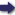(London :  Kegan Paul, Trench, Trübner & Co.,  1910.)

 Tools

## Search this bookPrev Page 40 Next``` ALBERUNTS INDIA. Simplifica¬ tion of the same method. Page 223. that this fraction and the other fractions which occur in the further course of this computation should have the same denominator. Dividing the universal iXnardtra days by the solar years of a kalpa, the quotient is the number of 'dnardtra days which belong to a solar year, viz. 5f3-|~|;-§w:T¥"ff days. Eeducing this fraction by the common divisor of 450,000, we get sa%i) days. The fraction may fur¬ ther be reduced by being divided by 3. The measures of solar and lunar years are about 360 days, as are also the civil years of sun and moon, the one being a little larger, the other a little shorter. The one of these measures, the lunar year, is used in this computation, whilst the other measure, the solar year, is sought for. The sum of the two quotients (of the middle and lower number) is the difference between the two kinds of years. The upper number is multiplied by the sum of the complete days, and the middle and lower numbers are multiplied by each of the two fractions. If we want to abbreviate the computation, and do not, like the Hindus, wish to find the mean motions of sun and moon, we add the two multiplicators of the middle and lower numbers together. This gives the sum of 10,220. To this sum we add, for the upper place, the product of the divisor x 10 = 96,000, and we get -^Vff-- Eeducing this fraction by the half, we get "-j^^V- In this chapter (p. 27) we have already explained that by multiplying the days by 5311, and dividing the product by 172,800, we get the number of the adhimdsas. If we now multiply the number of years instead of the days, the product is -r^^^j of the product which we should get when multiplying by the number of days. If we, therefore, want to have the same quotient which we get by the first division, we must divide by -y^-g- of the divisor by which we divided in the first case, viz. 480 (for 360 X 480 = 172,800). ```Prev Page 40 Next